# Lecture Notes in Deep Learning: Unsupervised Learning – Part 3

## Generative Adversarial Networks – The Basics

These are the lecture notes for FAU’s YouTube Lecture “Deep Learning“. This is a full transcript of the lecture video & matching slides. We hope, you enjoy this as much as the videos. Of course, this transcript was created with deep learning techniques largely automatically and only minor manual modifications were performed. Try it yourself! If you spot mistakes, please let us know!A common AI myth is that GANs are mainly used to worship cats. Image created using gifify. Source: YouTube

Welcome back to deep learning! So today, we finally want to look into the generative adversarial networks which are a key technology in unsupervised deep learning. So, let’s see what I have for you here.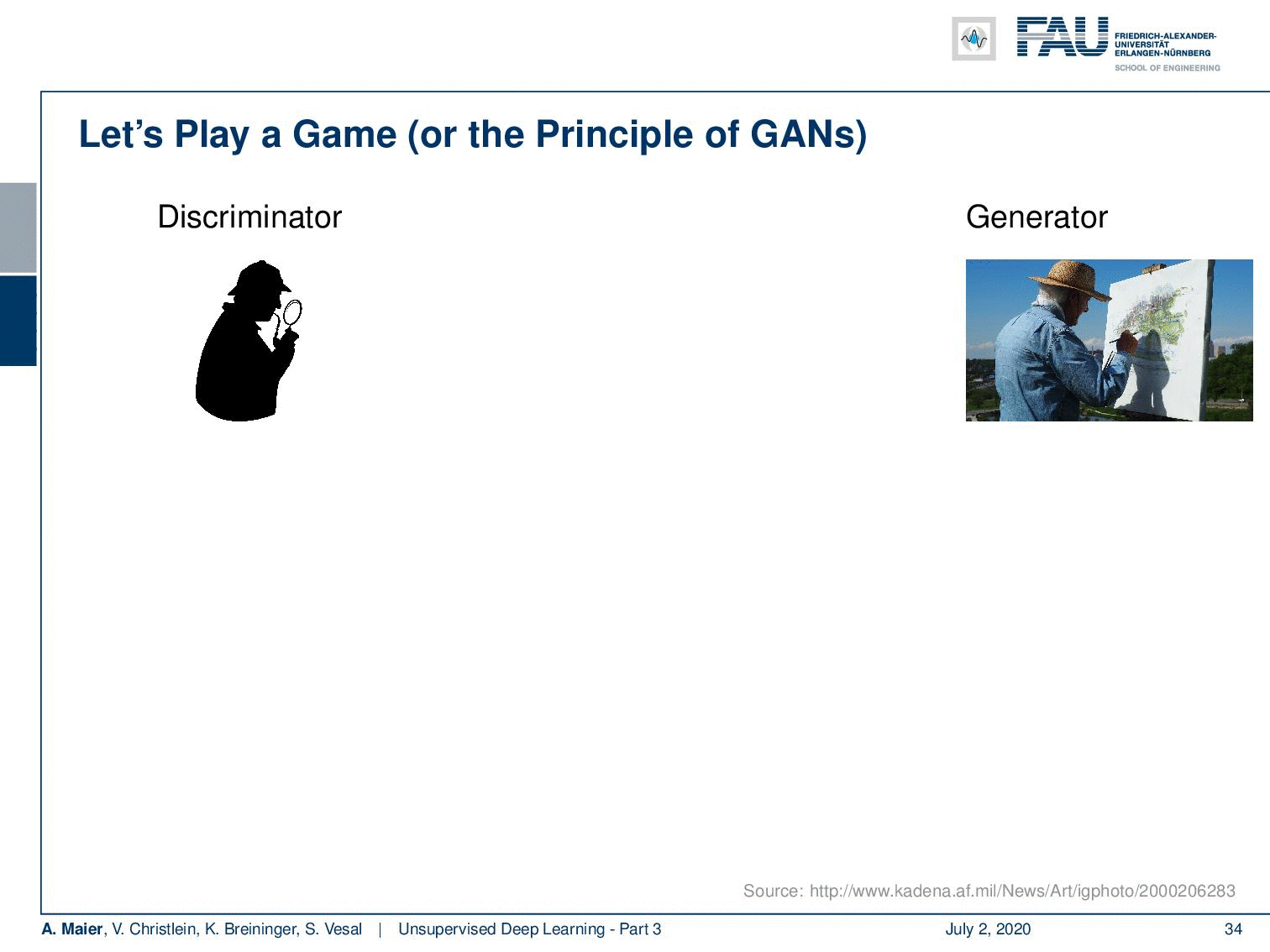The concept of GANs. Image under CC BY 4.0 from the Deep Learning Lecture.

Well, the unsupervised deep learning part generative adversarial networks come from the key idea that GANs to play the following game: You have a generator and a discriminator. Now the generator, one could argue is somebody who generates a fake image. Then, the discrimination has to figure out whether the generator actually produced something that’s real or something which is fake. So, the discriminator can decide fake or real and in order to train the discriminator, he has access to many real data observations. So, the outcome of the discriminator then is whether the input was real or fake. Well, of course, this is difficult to ask persons and artists to draw things. So, we replace the tool with deep neural networks and we have D that is the discriminator and we have G that is the generator. The generator receives some latent input some noise variable z and from the noise variable and the parameters, it produces some image. The discriminator then tries to figure out whether this was a real or fake image. So, the output of the discriminator is going to be 1 for real and 0 for fake.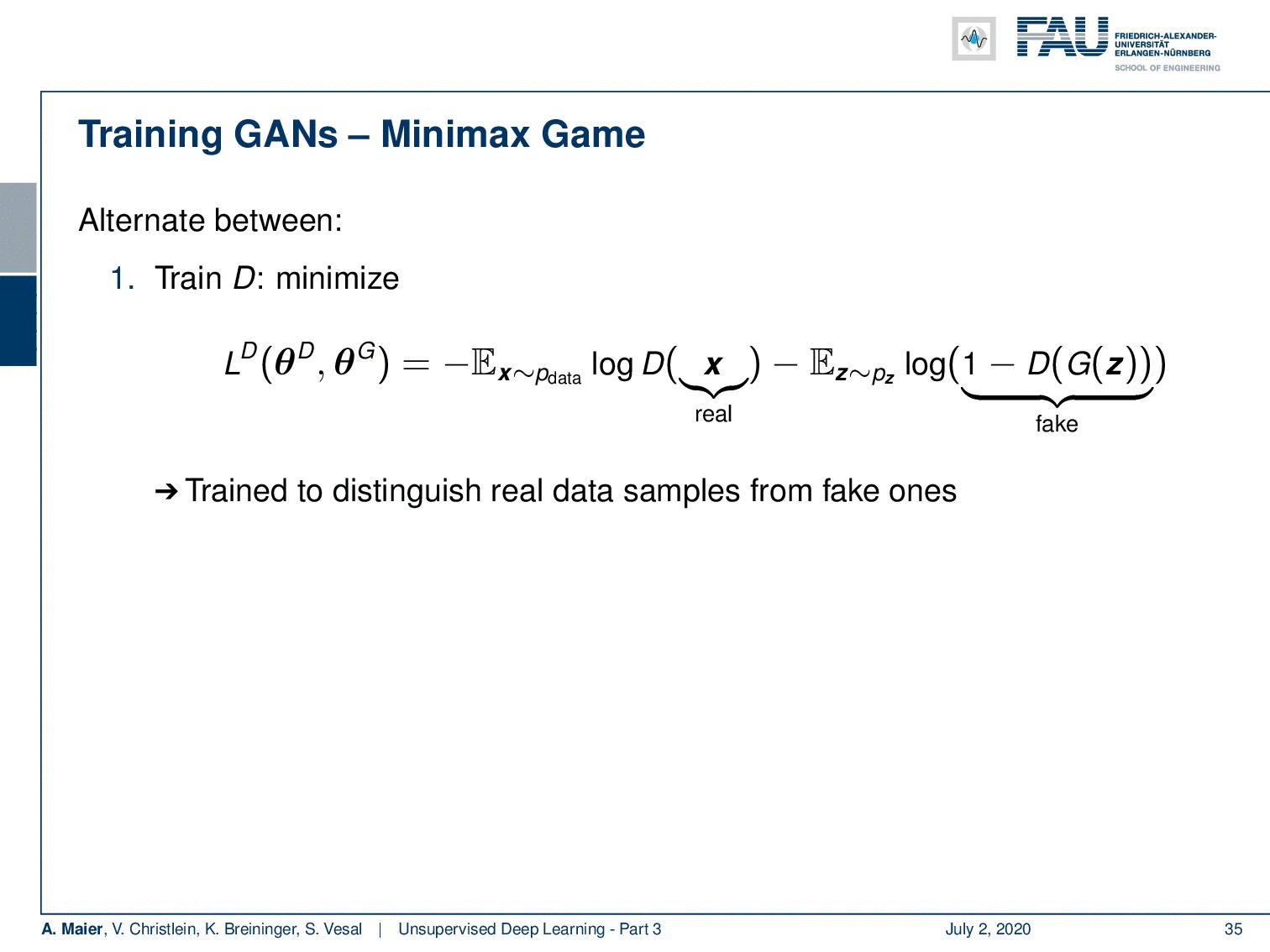The GAN loss. Image under CC BY 4.0 from the Deep Learning Lecture.

Once we have found this kind of neural network representation, we are also able to describe a loss. The loss of our discriminator is to minimize the following function that is dependent on the parameters of the discriminator and the parameters of the generator. It is essentially minimizing the expected value of x from the data. This is simply the logarithm of the output of our discriminator for real samples minus the expected value of some generated noise and that is the logarithm of 1 minus the discriminator of the generator of some noise. So, it’s trained to distinguish real data samples from fake ones. Now, if you want to train the generator you minimize the loss of the generator that is the negative loss of the discriminator. So, the generator minimizes the probability of the discriminator being correct. You train to generate domain images to fool D. Optionally, you can run k steps of one player for every step of the other player and the equilibrium is a saddle point of the discriminator loss.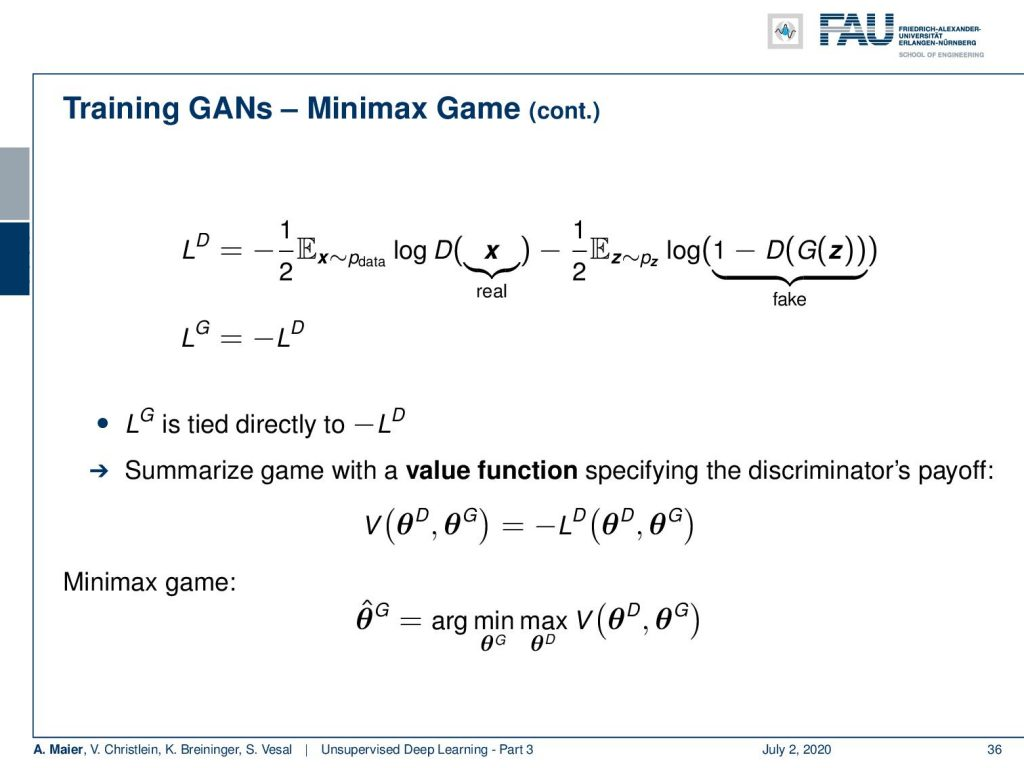GANs formulate their optimisation as a minimax game. Image under CC BY 4.0 from the Deep Learning Lecture.

If you look into this in more detail, you can find that the loss of the generator is directly tied to the negative loss of the discriminator. So, you can summarize this game with a value function specifying the discriminator’s payoff that is given as V. This is the negative loss of the discriminator and this then results in the following minimax game: So the optimal parameter set of the generator can be determined by maximizing V with respect to the discriminator nested into a minimization of the parameters of G with respect to the same value function.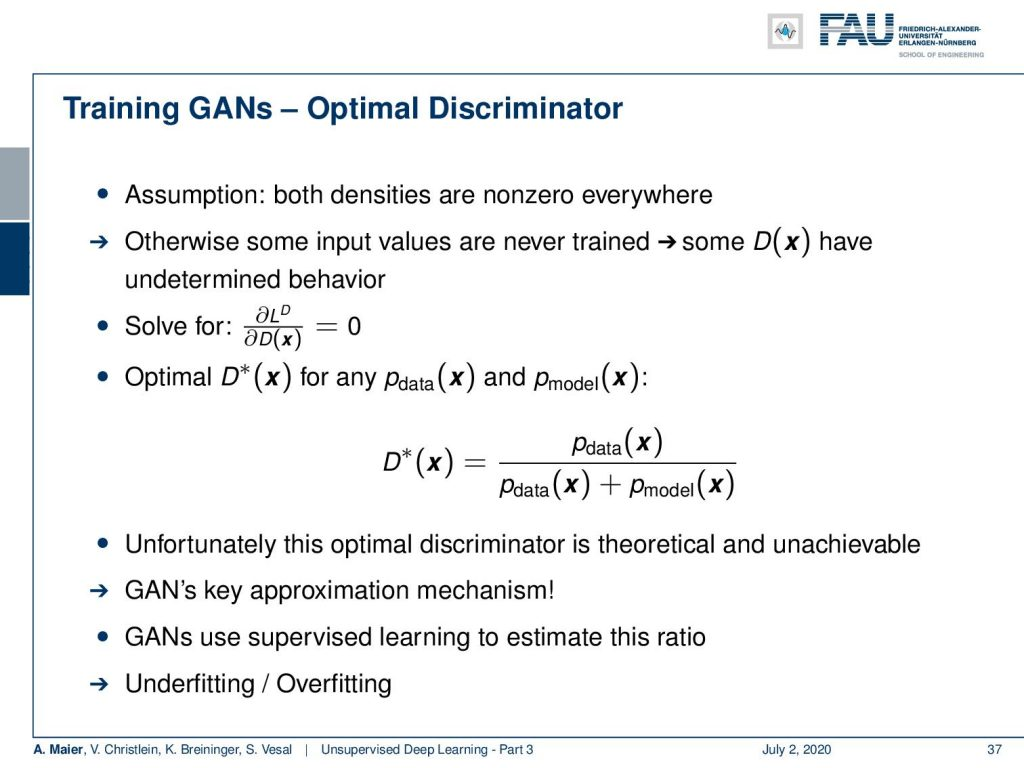The optimal discriminator can be found as a ratio of model and data. Image under CC BY 4.0 from the Deep Learning Lecture.

So, let’s have a look at the optimal discriminator. There a key assumption is that is both densities are nonzero everywhere. Otherwise, some input values would never be trained and the discriminator would have undetermined behavior in those areas. Then you solve with respect to the gradient of the discriminator loss with respect to the discriminator to be zero. You can find the optimal discriminator for any data distribution and any model distribution in the following way: the optimal discriminator is the distribution of the data divided by the distribution of the data plus the distribution of the model over your entire input domain of x. Unfortunately, this optimal discriminate is theoretical and unachievable. So, it’s key for GANs to have an estimation mechanism. You can use supervised learning to estimate this ratio. Then this leads to the problem of underfitting and overfitting.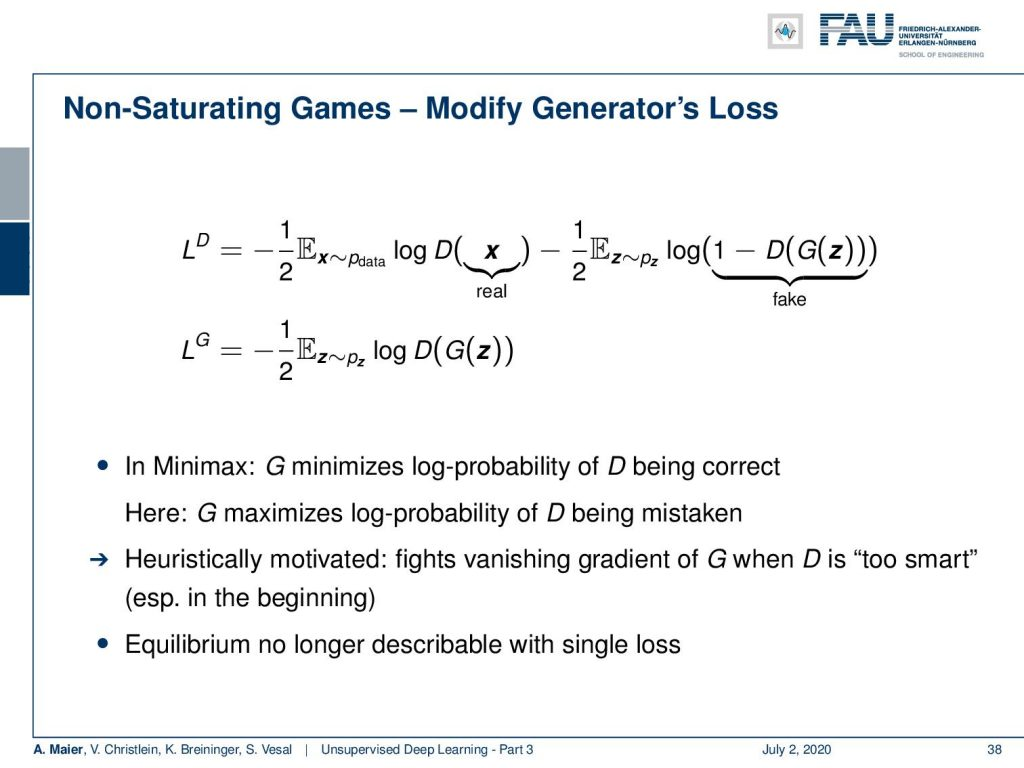This modified loss is non-saturating. Image under CC BY 4.0 from the Deep Learning Lecture.

Now, what else can we do? We can do non-saturating games where we modify the generator’s loss. Then, in this example, we are no longer using the same function for both. Instead, we have a new loss for the generator where we simply compute the expected value of the logarithm of the discriminator of the generator given some input noise. In minimax, G minimizes the log probability of D being correct. In this solution, G minimizes the log probability of D being mistaken. It’s heuristically motivated because it fights the vanishing gradient of G when D is too smart. This is particularly a problem in the beginning. However, the equilibrium is no longer describable using a single loss.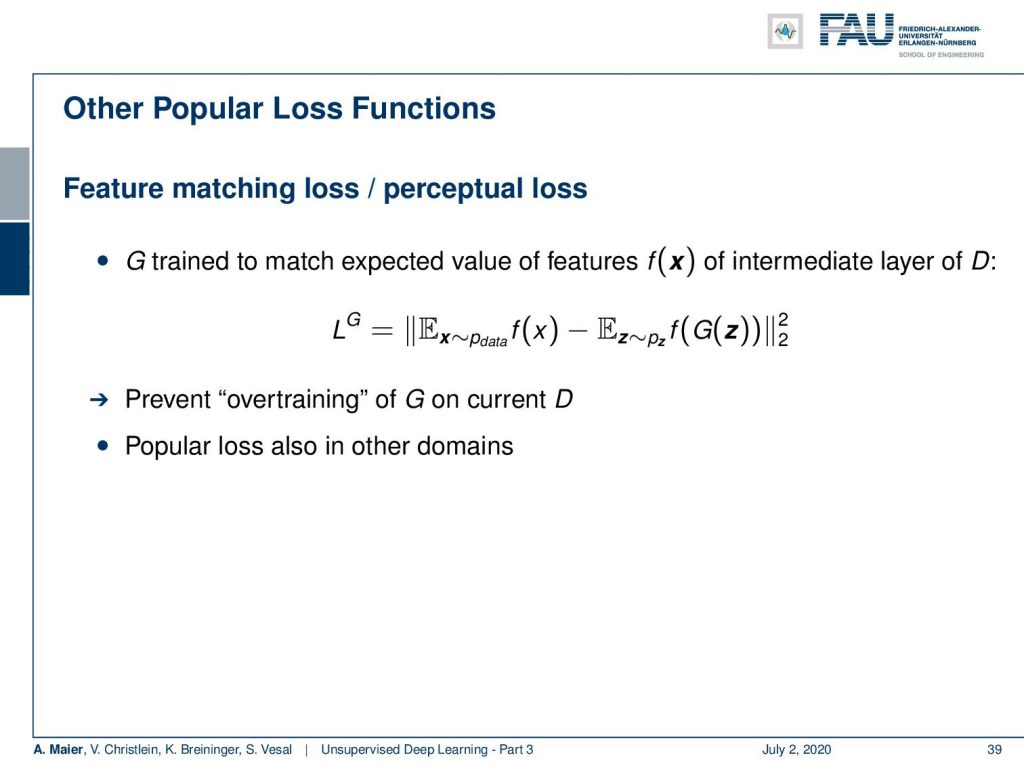Other variants of the GAN loss. Image under CC BY 4.0 from the Deep Learning Lecture.

So, there are a lot of things like extensions that are quite popular like the feature matching loss or the perceptual loss. Here, G is trying to match the expected value of features f(x) of some intermediate layer of D. You’ve seen this already that f can be for example some other network and some layer 3 or layer 5 representation. Then, you want the expected values of these representations to be the same given for real inputs as well as for generated noise images. So, here you want to prevent the overtraining of the generator on the current discriminator. By the way, this is also a popular loss in many other domains.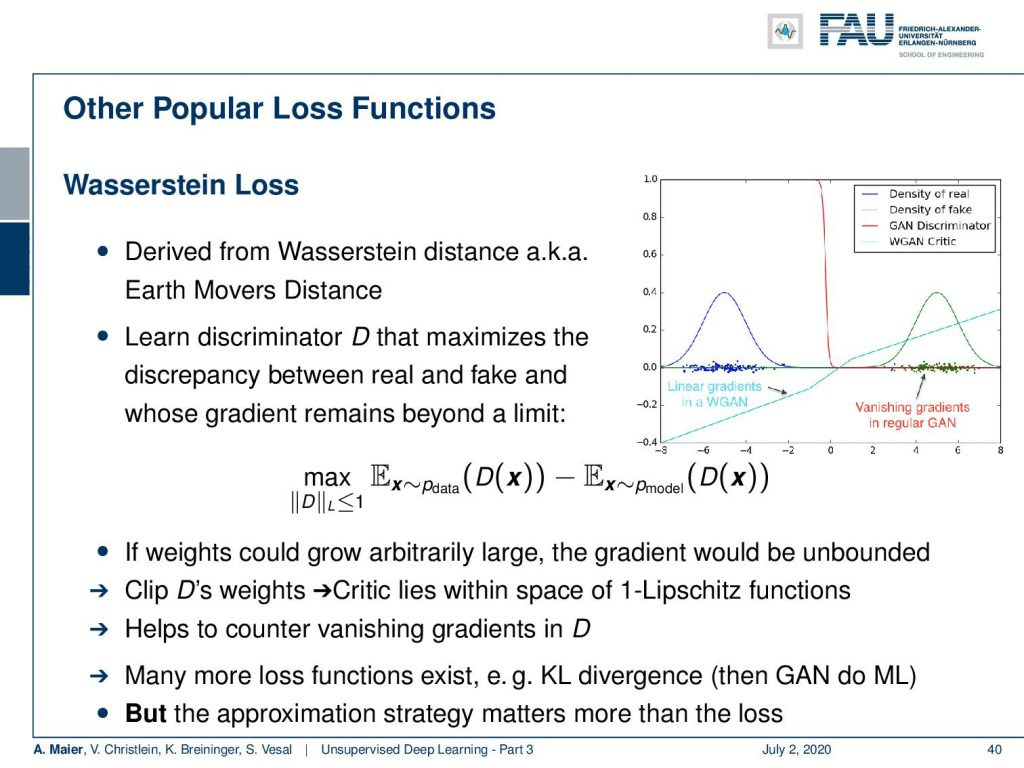The Wasserstein loss. Image under CC BY 4.0 from the Deep Learning Lecture.

What else can be done? Well, there’s the so-called Wasserstein loss. It’s derived from the Wasserstein distance which is also known as the earth movers distance. Here, you learn a discriminator that maximizes the discrepancy between the real and fake samples, and at the same time, you restrict the gradient to stay behind a certain limit. So, you essentially limit the gradient towards a specific Lipschitz constant which is the maximum slope of the gradient. Here, in the image on the right-hand side, you can see that out of the red discrimination curve which saturated very quickly, you can then create a discriminator that has this non-saturated loss. This way, you will always be able to find good gradients, even in areas where you’re already saturated with your discriminator. Again, this helps to counter vanishing gradients in the discriminator. Many more loss functions exist like the KL divergence. Then, the GANs actually do maximum likelihood, but the approximation strategy matters much more than the loss.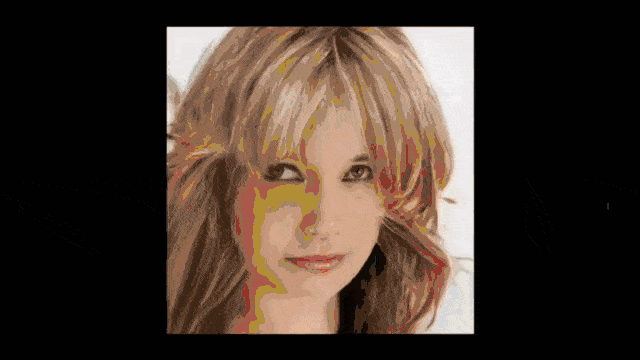Faces can be generated extremely well with GANS. Image created using gifify. Source: YouTube

So, how do we evaluate GANs? Well, we can, of course, look at the image and say: “Yeah, they look realistic! Or not?” But this is kind of intractable for large data sets. So, you have to use a score for images. One idea is the inception score.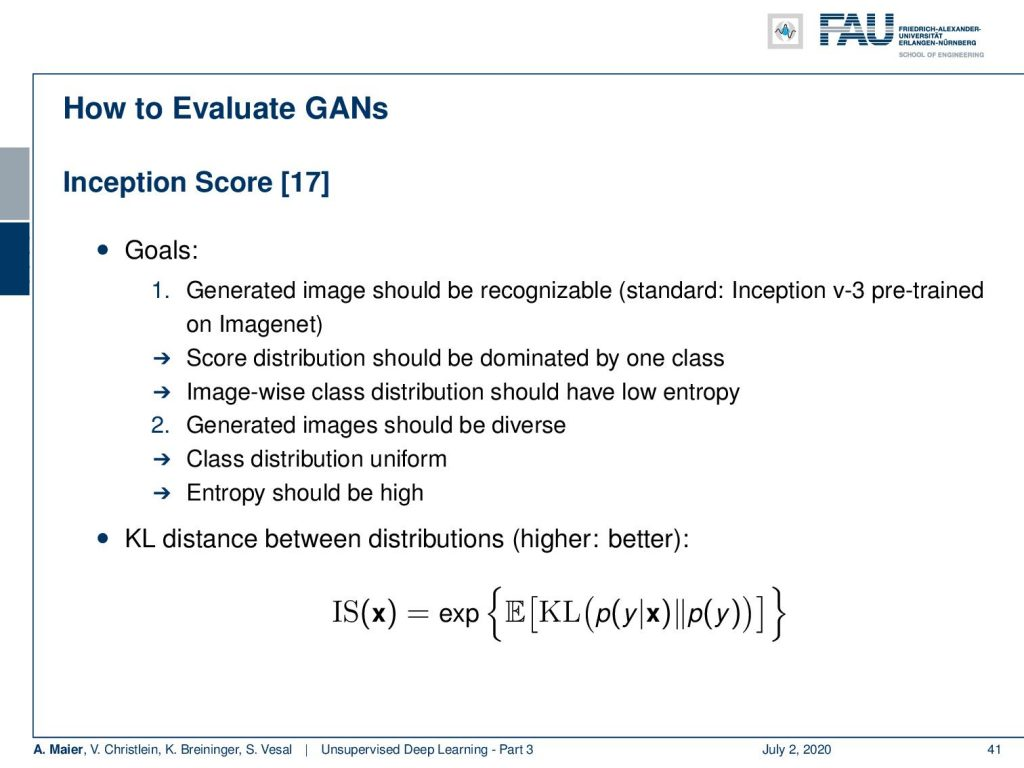Evaluation of GANs using the Inception Score. Image under CC BY 4.0 from the Deep Learning Lecture.

The inception score is based on two goals. One goal is that the generated images should be recognizable. So, you use, for example, an Inception v3 pre-trained Network on ImageNet and you want the score distribution to be dominated by one class. The image-wise class distribution should have low entropy. At the same time, you want the generated images to be diverse. So the overall class distribution should be more or less uniform. The entropy should be high. So, you can then express this inception score as e to the power of the expected value of the KL divergence between p(y|x) and p(y).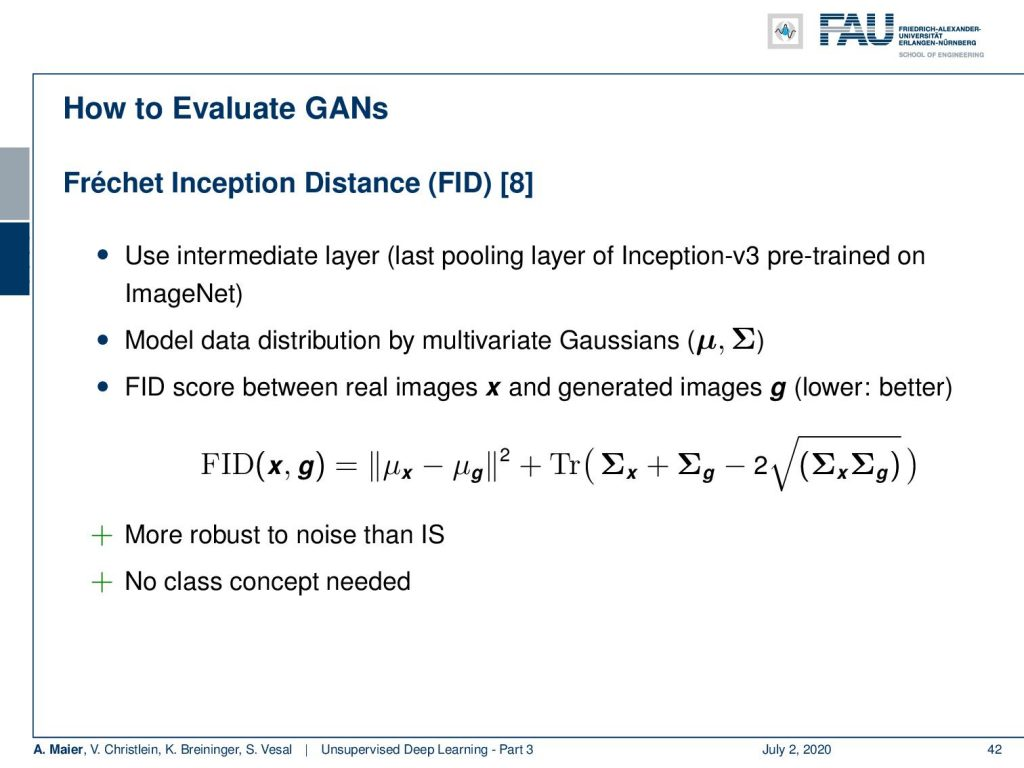The Fréchet Inception Score. Image under CC BY 4.0 from the Deep Learning Lecture.

Another measurement is the Fréchet inception distance which is using an intermediate layer. So, the last pooling layer of Inception v3 pretrained on ImageNet, for example. Then, you model the data distribution by multivariate Gaussians. The FID score between the real images x and the generated images g can be expressed as the difference between the mean values of x and g in an l2 norm plus the trace of the covariance matrices of x and g minus two times the square root of covariance matrix x times covariance matrix g. This is more robust than the inception score. We don’t need the class concept. In this case, we can simply work on multivariate Gaussians in order to model the distributions.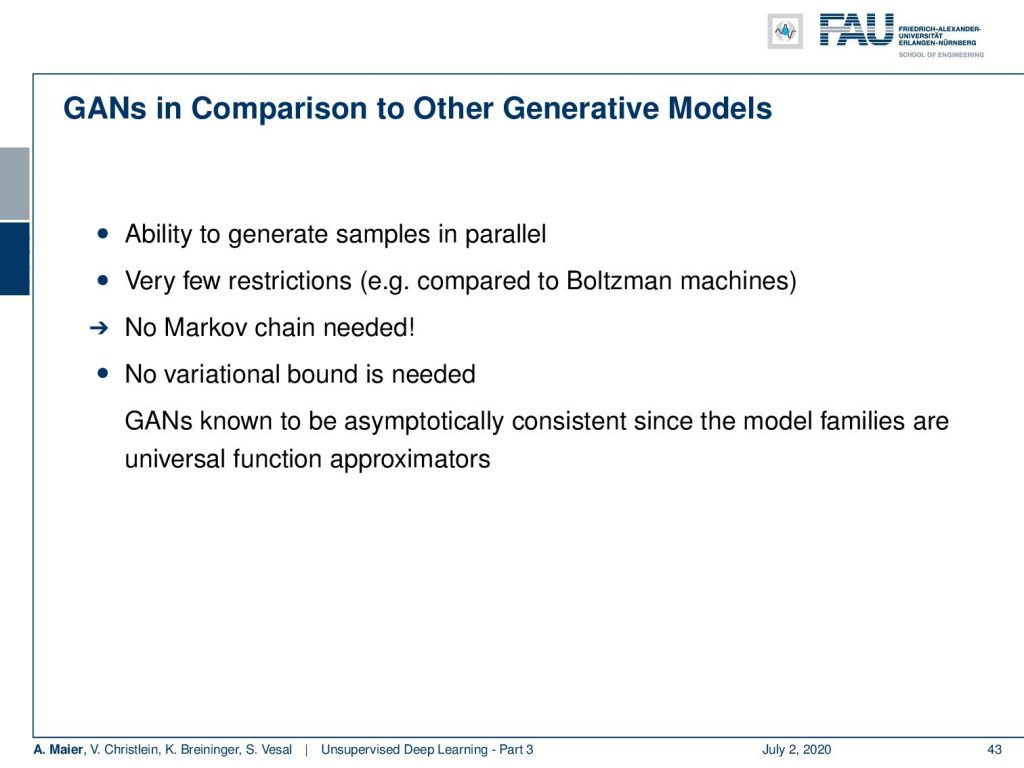Advantages of GANs. Image under CC BY 4.0 from the Deep Learning Lecture.

A big advantage of GANs is that they are able to generate samples in parallel. There are very few restrictions. For example, compared to the Boltzmann machines that have plenty of restrictions: You don’t need a Markov chain in this model. There are also no variational bounds needed. GANs are known to be asymptotically consistent since the model families are universal function approximators. So, this was a very first introduction to GANs.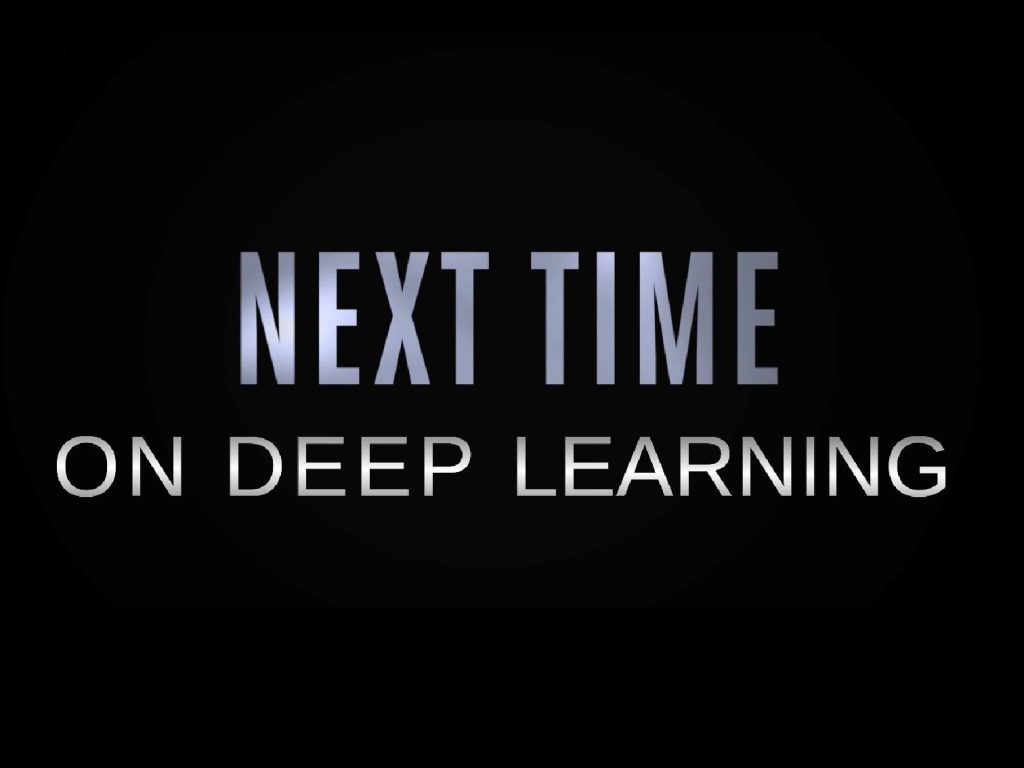More exciting things coming up in this deep learning lecture. Image under CC BY 4.0 from the Deep Learning Lecture.

In the next video, we want to talk a bit about more advanced GAN concepts like the conditional GANs where we can also start and modeling constraints and conditions into the generation process. People also looked into a very cool technique that is called the cycle GAN which allows unpaired to domain translation. So, you can translate images from day to night. You can even translate horses to zebras and zebras to horses. So, a very, very cool technique coming up. I hope you enjoyed this video and I’m looking forward to seeing you in the next one. Thank you very much!

If you liked this post, you can find more essays here, more educational material on Machine Learning here, or have a look at our Deep LearningLecture. I would also appreciate a follow on YouTubeTwitterFacebook, or LinkedIn in case you want to be informed about more essays, videos, and research in the future. This article is released under the Creative Commons 4.0 Attribution License and can be reprinted and modified if referenced. If you are interested in generating transcripts from video lectures try AutoBlog.

Link – Variational Autoencoders:
Link – NIPS 2016 GAN Tutorial of Goodfellow
Link – How to train a GAN? Tips and tricks to make GANs work (careful, not
everything is true anymore!)
Link - Ever wondered about how to name your GAN?

## References

 Xi Chen, Xi Chen, Yan Duan, et al. “InfoGAN: Interpretable Representation Learning by Information Maximizing Generative Adversarial Nets”. In: Advances in Neural Information Processing Systems 29. Curran Associates, Inc., 2016, pp. 2172–2180.
 Pascal Vincent, Hugo Larochelle, Isabelle Lajoie, et al. “Stacked denoising autoencoders: Learning useful representations in a deep network with a local denoising criterion”. In: Journal of Machine Learning Research 11.Dec (2010), pp. 3371–3408.
 Emily L. Denton, Soumith Chintala, Arthur Szlam, et al. “Deep Generative Image Models using a Laplacian Pyramid of Adversarial Networks”. In: CoRR abs/1506.05751 (2015). arXiv: 1506.05751.
 Richard O. Duda, Peter E. Hart, and David G. Stork. Pattern classification. 2nd ed. New York: Wiley-Interscience, Nov. 2000.
 Asja Fischer and Christian Igel. “Training restricted Boltzmann machines: An introduction”. In: Pattern Recognition 47.1 (2014), pp. 25–39.
 John Gauthier. Conditional generative adversarial networks for face generation. Mar. 17, 2015. URL: http://www.foldl.me/2015/conditional-gans-face-generation/ (visited on 01/22/2018).
 Ian Goodfellow. NIPS 2016 Tutorial: Generative Adversarial Networks. 2016. eprint: arXiv:1701.00160.
 Martin Heusel, Hubert Ramsauer, Thomas Unterthiner, et al. “GANs Trained by a Two Time-Scale Update Rule Converge to a Local Nash Equilibrium”. In: Advances in Neural Information Processing Systems 30. Curran Associates, Inc., 2017, pp. 6626–6637.
 Geoffrey E Hinton and Ruslan R Salakhutdinov. “Reducing the dimensionality of data with neural networks.” In: Science 313.5786 (July 2006), pp. 504–507. arXiv: 20.
 Geoffrey E. Hinton. “A Practical Guide to Training Restricted Boltzmann Machines”. In: Neural Networks: Tricks of the Trade: Second Edition. Berlin, Heidelberg: Springer Berlin Heidelberg, 2012, pp. 599–619.
 Phillip Isola, Jun-Yan Zhu, Tinghui Zhou, et al. “Image-to-Image Translation with Conditional Adversarial Networks”. In: (2016). eprint: arXiv:1611.07004.
 Diederik P Kingma and Max Welling. “Auto-Encoding Variational Bayes”. In: arXiv e-prints, arXiv:1312.6114 (Dec. 2013), arXiv:1312.6114. arXiv: 1312.6114 [stat.ML].
 Jonathan Masci, Ueli Meier, Dan Ciresan, et al. “Stacked Convolutional Auto-Encoders for Hierarchical Feature Extraction”. In: Artificial Neural Networks and Machine Learning – ICANN 2011. Berlin, Heidelberg: Springer Berlin Heidelberg, 2011, pp. 52–59.
 Luke Metz, Ben Poole, David Pfau, et al. “Unrolled Generative Adversarial Networks”. In: International Conference on Learning Representations. Apr. 2017. eprint: arXiv:1611.02163.
 Mehdi Mirza and Simon Osindero. “Conditional Generative Adversarial Nets”. In: CoRR abs/1411.1784 (2014). arXiv: 1411.1784.
 Alec Radford, Luke Metz, and Soumith Chintala. Unsupervised Representation Learning with Deep Convolutional Generative Adversarial 2015. eprint: arXiv:1511.06434.
 Tim Salimans, Ian Goodfellow, Wojciech Zaremba, et al. “Improved Techniques for Training GANs”. In: Advances in Neural Information Processing Systems 29. Curran Associates, Inc., 2016, pp. 2234–2242.
 Andrew Ng. “CS294A Lecture notes”. In: 2011.
 Han Zhang, Tao Xu, Hongsheng Li, et al. “StackGAN: Text to Photo-realistic Image Synthesis with Stacked Generative Adversarial Networks”. In: CoRR abs/1612.03242 (2016). arXiv: 1612.03242.
 Han Zhang, Tao Xu, Hongsheng Li, et al. “Stackgan: Text to photo-realistic image synthesis with stacked generative adversarial networks”. In: arXiv preprint arXiv:1612.03242 (2016).
 Bolei Zhou, Aditya Khosla, Agata Lapedriza, et al. “Learning Deep Features for Discriminative Localization”. In: 2016 IEEE Conference on Computer Vision and Pattern Recognition (CVPR). Las Vegas, June 2016, pp. 2921–2929. arXiv: 1512.04150.
 Jun-Yan Zhu, Taesung Park, Phillip Isola, et al. “Unpaired Image-to-Image Translation using Cycle-Consistent Adversarial Networks”. In: CoRR abs/1703.10593 (2017). arXiv: 1703.10593.